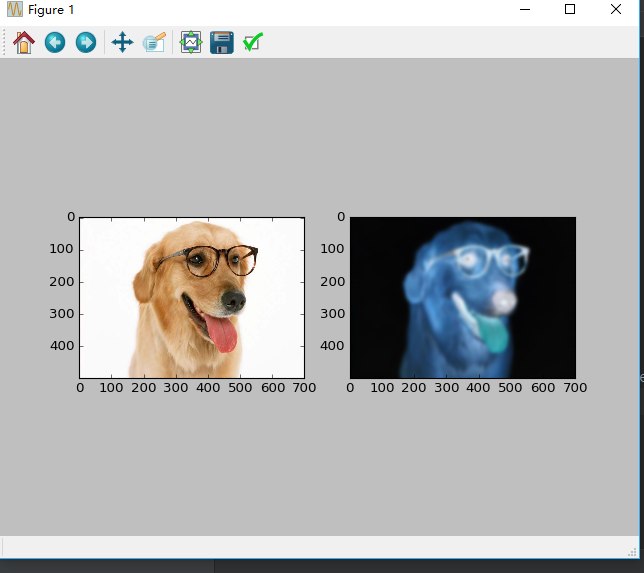2个回答

from PIL import Image
from pylab import *
from numpy import *
from scipy import ndimage
from scipy.ndimage import filters
from scipy import misc

im = array(Image.open('1.jpg'))
im2 = zeros(im.shape)
m,n,p = im.shape

for i in range(p):
im2[:,:,i] = filters.gaussian_filter(im[:,:,i],5)
im2 = uint8(im2)
subplot(1,2,1)
imshow(im)

subplot(1,2,2)
imshow(im2)

show()